Tait equation of state

(diff) ← Older revision | Latest revision (diff) | Newer revision → (diff)

The Tait equation is an equation of state. The equation was originally published by Peter Guthrie Tait in 1888. (Yuan-Hui Li, 15 May 1967, Equation of State of Water and Sea Water, Journal of Geophysical Research 72 (10), p. 2665.) It is sometimes written as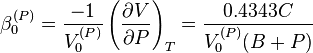$\beta_0^{(P)} = \frac{-1}{V_0^{(P)}} \left ( \frac{\partial V}{\partial P} \right )_T = \frac{0.4343C}{V_0^{(P)}(B+P)}$

or in the integrated form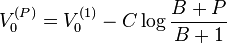$V_0^{(P)} = V_0^{(1)} - C \log \frac{B+P}{B+1}$

where

•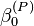$\beta_0^{(P)}$ is the compressibility of water.
•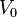$V_0 \$ is the specific volume of water
•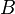$B \$ and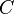$C \$ are functions of temperature that are independent of pressure.